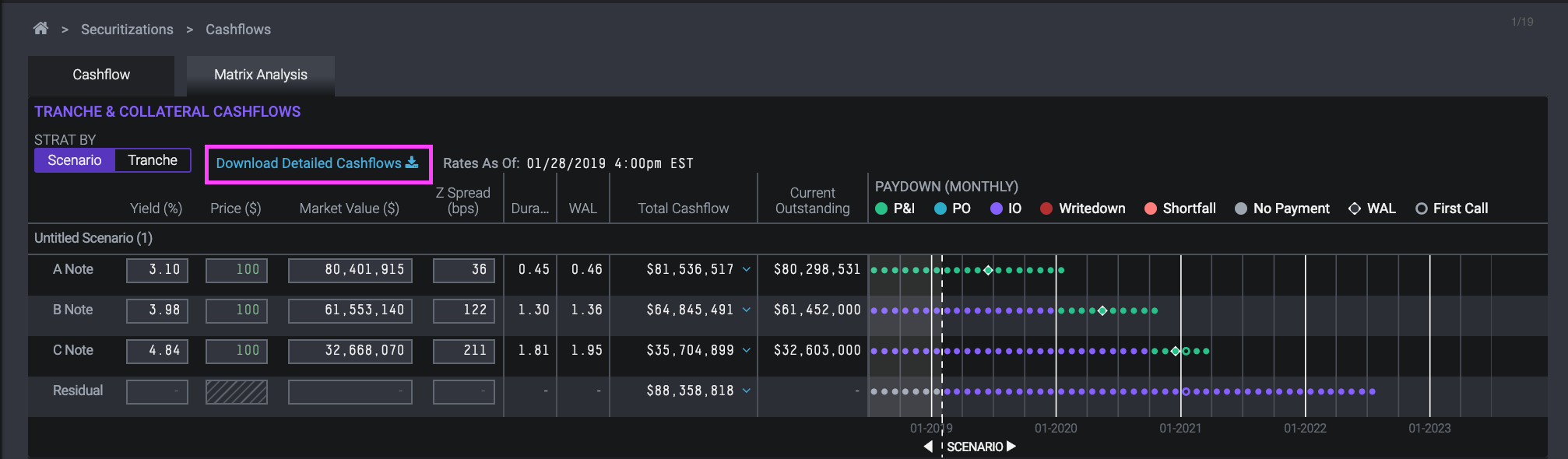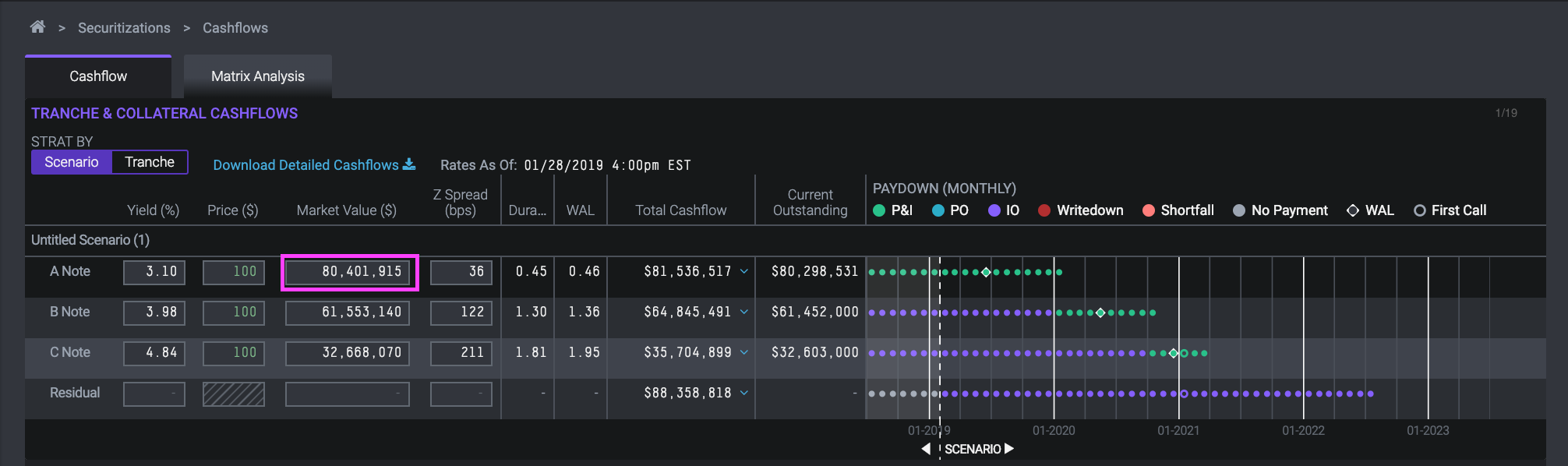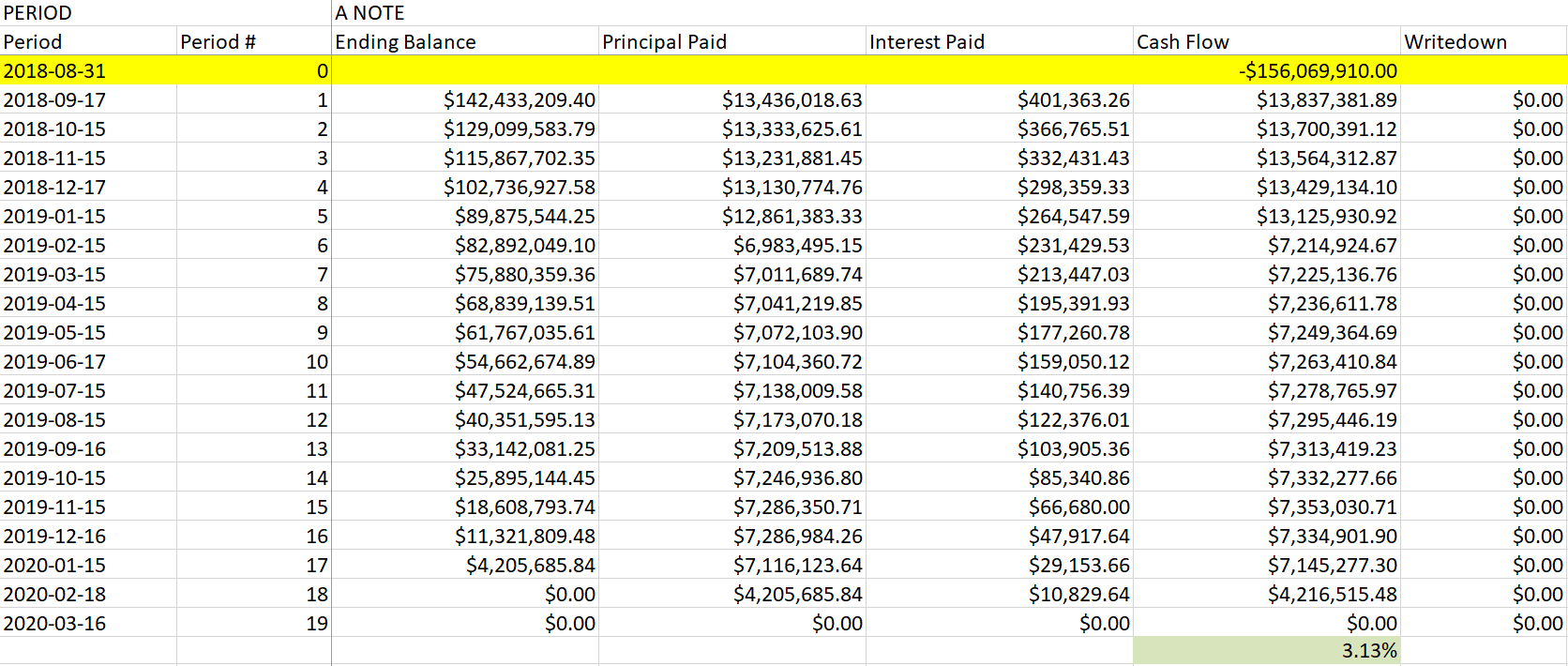The yield dv01 generates when pricing cash flows is bond equivalent yield (BEY). BEY is the industry standard for quoting bond yields. You can recreate the BEY in Excel using the cash flow output from a given scenario.

General yield formulas like Excel's XIRR use a pricing convention like ACT/365. This results in slight differences when trying to recreate yield values manually, but they can be easily be converted. Below we'll go through an example.

Consider the deal AVNT 2018-A run at 5 CPR, 5 CDR, and 100 SEV, with a settle date of 8/31/18:At a price of 100, you can see the yield for the A, B and C Note is 3.10%, 3.98% and 4.84%, respectively.You can extract the tranche level cash flows by clicking “Download Detailed Cashflows”.Since yield is a price concept, we have to add in the market value of the bond as a cash outflow in period 0. In the Excel spreadsheet, we need to add a row just above period 1, to represent the cash outflow for the respective bond. For the A note, the outflow is \$80,401,915, the market value of the bond.The market value for the respective note should be added as a negative cash flow in period zero. It's important to use the market value as it includes accrued interest that needs to be considered when calculating yield. XIRR can then be calculated with the inputs XIRR(cash flow, periods).Using the cash flows above, the yield comes out to 3.13% for the A tranche. However, the day convention used by Excel's XIRR formula is ACT/365, while dv01 uses a bond equivalent yield. To convert between the two, use the formula:

`2*((1+[XIRR])^0.5)-2`

In the case of the A tranche, the BEY would be calculated as follows:

`2*((1+0.03131825)^0.5)-2 == 3.10%`

There may be a slight difference in rate with what is displayed on the website due to cash flow values being rounded to two decimal places when exported to Excel.